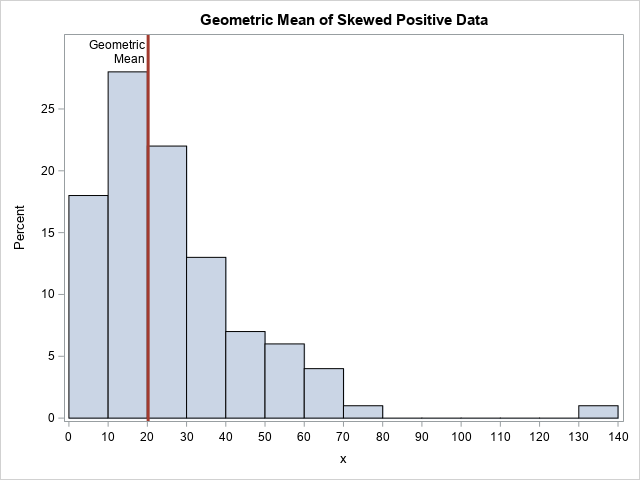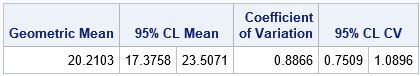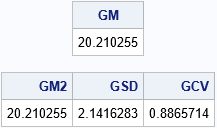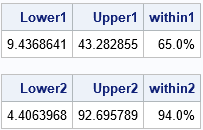I frequently see questions on SAS discussion forums about how to compute the geometric mean and related quantities in SAS. Unfortunately, the answers to these questions are sometimes confusing or even wrong. In addition, some published papers and web sites that claim to show how to calculate the geometric mean in SAS contain wrong or misleading information.

This article shows how to compute the geometric mean, the geometric standard deviation, and the geometric coefficient of variation in SAS. It first shows how to use PROC TTEST to compute the geometric mean and the geometric coefficient of variation. It then shows how to compute several geometric statistics in the SAS/IML language.

For an introduction to the geometric mean, see "What is a geometric mean." For information about the (arithmetic) coefficient of variation (CV) and its applications, see the article "What is the coefficient of variation?"

### Compute the geometric mean and geometric CV in SAS

As discussed in my previous article, the geometric mean arises naturally when positive numbers are being multiplied and you want to find the average multiplier. Although the geometric mean can be used to estimate the "center" of any set of positive numbers, it is frequently used to estimate average values in a set of ratios or to compute an average growth rate.

The TTEST procedure is the easiest way to compute the geometric mean (GM) and geometric CV (GCV) of positive data. To demonstrate this, the following DATA step simulates 100 random observations from a lognormal distribution. PROC SGPLOT shows a histogram of the data and overlays a vertical line at the location of the geometric mean.

```%let N = 100; data Have; call streaminit(12345); do i = 1 to &N; x = round( rand("LogNormal", 3, 0.8), 0.1); /* generate positive values */ output; end; run;   title "Geometric Mean of Skewed Positive Data"; proc sgplot data=Have; histogram x / binwidth=10 binstart=5 showbins; refline 20.2 / axis=x label="Geometric/Mean" splitchar="/" labelloc=inside lineattrs=GraphData2(thickness=3); xaxis values=(0 to 140 by 10); yaxis offsetmax=0.1; run;```Where is the "center" of these data? That depends on your definition. The mode of this skewed distribution is close to x=15, but the arithmetic mean is about 26.4. The mean is pulled upwards by the long right tail. It is a mathematical fact that the geometric mean of data is always less than the arithmetic mean. For these data, the geometric mean is 20.2.

To compute the geometric mean and geometric CV, you can use the DIST=LOGNORMAL option on the PROC TTEST statement, as follows:

```proc ttest data=Have dist=lognormal; var x; ods select ConfLimits; run;```The geometric mean, which is 20.2 for these data, estimates the "center" of the data. Notice that the procedure does not report the geometric standard deviation (or variance), but instead reports the geometric coefficient of variation (GCV), which has the value 0.887 for this example. The documentation for the TTEST procedure explains why the GCV is the better measure of variation: "For lognormal data, the CV is the natural measure of variability (rather than the standard deviation) because the CV is invariant to multiplication of [the data]by a constant."

You might wonder whether data need to be lognormally distributed to use this table. The answer is that the data do not need to be lognormally distributed to use the geometric mean and geometric CV. However, the 95% confidence intervals for these quantities assume log-normality.

### Definitions of geometric statistics

As T. Kirkwood points out in a letter to the editors of Biometric (Kirkwood, 1979), if data are lognormally distributed as LN(μ σ), then

• The quantity GM = exp(μ) is the geometric mean. It is estimated from a sample by the quantity exp(m), where m is the arithmetic mean of the log-transformed data.
• The quantity GSD = exp(σ) is defined to be the geometric standard deviation. The sample estimate is exp(s), where s is the standard deviation of the log-transformed data.
• The geometric standard error (GSE) is defined by exponentiating the standard error of the mean of the log-transformed data. Geometric confidence intervals are handled similarly.
• Kirkwood's proposal for the geometric coefficient of variation (GCV) is not generally used. Instead, the accepted definition of the GCV is GCV = sqrt(exp(σ2) – 1), which is the definition that is used in SAS. The estimate for the GCV is sqrt(exp(s2) – 1).

You can use these formulas to compute the geometric statistics for any positive data. However, only for lognormal data do the statistics have a solid theoretical basis: transform to normality, compute a statistic, apply the inverse transform.

### Compute the geometric mean in SAS/IML

You can use the SAS/IML language to compute the geometric mean and other "geometric statistics" such as the geometric standard deviation and the geometric CV. The GEOMEAN function is a built-in SAS/IML function, but the other statistics are implemented by explicitly computing statistics of the log-transformed data, as described in the previous section:

```proc iml; use Have; read all var "x"; close; /* read in positive data */ GM = geomean(x); /* built-in GEOMEAN function */ print GM;   /* To estimate the geometric mean and geometric StdDev, compute arithmetic estimates of log(X), then EXP transform the results. */ n = nrow(x); z = log(x); /* log-transformed data */ m = mean(z); /* arithmetic mean of log(X) */ s = std(z); /* arithmetic std dev of log(X) */ GM2 = exp(m); /* same answer as GEOMEAN function */ GSD = exp(s); /* geometric std dev */ GCV = sqrt(exp(s**2) - 1); /* geometric CV */ print GM2 GSD GCV;```Note that the GM and GCV match the output from PROC TTEST.

What does the geometric standard deviation mean? As for the arithmetic mean, you need to start by thinking about the location of the geometric mean (20.2). If the data are normally distributed, then about 68% of the data are within one standard deviation of the mean, which is the interval [m0s, m+s]. For lognormal data, about 68% of the data should be in the interval [GM/GSD, GM*GSD] and, in fact, 65 out of 100 of the simulated observations are in that interval. Similarly, about 95% of lognormal data should be in the interval [GM/GSD2, GM*GSD2]. For the simulated data, 94 out of 100 observations are in the interval, as shown below:I am not aware of a similar interpretation of the geometric coefficient of variation. The GCV is usually used to compare two samples. As opposed to the confidence intervals in the previous paragraph, the GCV does not make any reference to the geometric mean of the data.

### Other ways to compute the geometric mean

The methods in this article are the simplest ways to compute the geometric mean in SAS, but there are other ways.

• You can use the DATA step to log-transform the data, use PROC MEANS to compute the descriptive statistics of the log-transformed data, then use the DATA step to exponentiate the results.
• PROC SURVEYMEANS can compute the geometric mean (with confidence intervals) and the standard error of the geometric mean for survey responses. However, the variance of survey data is not the same as the variance of a random sample, so you should not use the standard error statistic unless you have survey data.

As I said earlier, there is some bad information out there on the internet about this topic, so beware. A site that seems to get all the formulas correct and present the information in a reasonable way is Alex Kritchevsky's blog.

You can download the complete SAS program that I used to compute the GM, GSD, and GCV. The program also shows how to compute confidence intervals for these quantities.

The post Compute the geometric mean, geometric standard deviation, and geometric CV in SAS appeared first on The DO Loop.